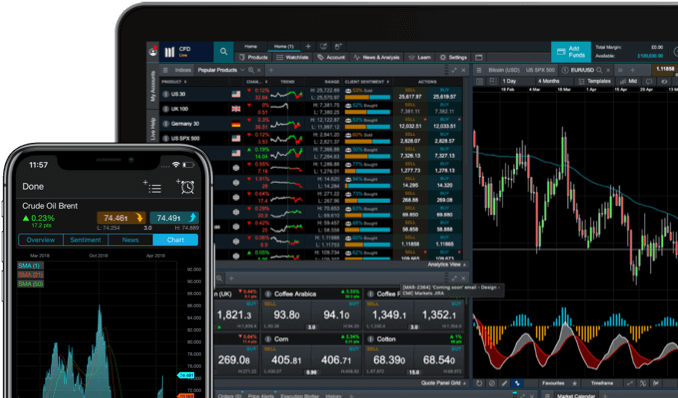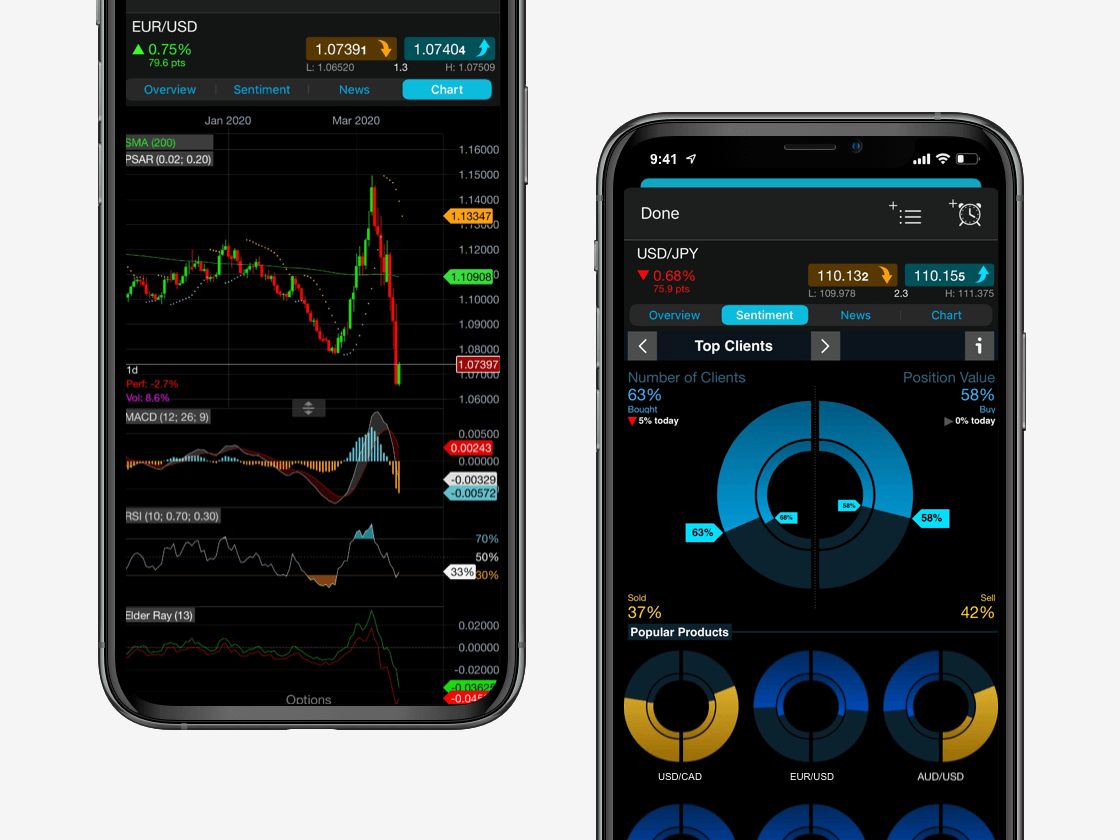Spread bets and CFDs are complex instruments and come with a high risk of losing money rapidly due to leverage. 78% of retail investor accounts lose money when spread betting and/or trading CFDs with this provider. You should consider whether you understand how spread bets and CFDs work and whether you can afford to take the high risk of losing your money.

# Sharpe ratio

The Sharpe ratio (or Sharpe Index) is named after its creator William Sharpe, the 1990 winner of the Nobel Prize in economic sciences. It is a measure of investment portfolio performance. The Sharpe ratio represents the return of a portfolio, without taking into account the “risk-free” interest rate and indicates the return percentage for each risk unit carried by the investment.

Using the Sharpe ratio as part of your fundamental analysis strategy​ helps to provide important financial information that can be used to make smarter trading decisions.

See inside our platform## What is the Sharpe ratio?

The Sharpe ratio is defined as the measure of the risk-adjusted return of a financial portfolio and is used to help investors understand the return of an investment compared to its risk. The measure assesses how much risk a trader has taken or is willing to take to generate those returns, otherwise known as the risk/reward ratio​. Investors are supposed to be compensated for taking extra risk beyond holding risk-free assets.

The Sharpe ratio can be easily applied to any time series of returns without the need for additional information regarding the source of volatility and/or profitability. It is usually used to compare the risk-adjusted returns of different kind of investments like shares, ETFs, mutual funds, and investment portfolios.

### Sharpe ratio vs Treynor ratio

The Treynor ratio​ is based on the same logic as the Sharpe ratio; however, when measuring risk level, it uses as a parameter the beta coefficient​ (instead of the standard deviation), which instead of measuring the total risk taken by the investor, only measures the systematic risk, i.e. the fund sensitivity to market movements and fluctuations.

Financial assets that have a beta greater than one are considered more sensitive, and therefore involve a higher level of risk than the market average; on the contrary, financial assets with a beta less than one are considered less risky than the market average. This choice is justified by the fact that specific risk can be eliminated through good diversification practices, therefore, performance assessment must be based solely on the risk component actually presented in the portfolio.

### Sharpe ratio vs Sortino ratio

The difference between these two risk measures is essentially that the Sortino ratio focuses more heavily on the swings and fluctuations occurring above or below an excess return. Risk essentially revolves around the fact that the threshold rate of return desired by the investor might not be reached. The Sharpe ratio on the other hand, focuses more on variability and on the risk/return ratio.

From this point of view, it can be stated that these two indices can be used together. The Sharpe ratio can be used as the primary tool and, then the Sortino ratio can be used to analyse and make a selection between two investments that have a fairly similar Sharpe ratio.

In closing, it might be useful to remember to not rely excessively on these indicators, because although they are important, they can also lead investors to the wrong conclusions. Usually, analysts prefer to use the Sharpe ratio to measure low-volatility investment portfolios and the Sortino ratio to measure high-volatility​​ investment portfolios.

## Sharpe ratio example

Let’s compare two funds, which during a certain period, earn a 20% and a 25% return respectively. The first one yields a 20% return while enjoying a stable and regular trend, whereas the second one reaches a 25% return while facing a series of strong fluctuations. The first fund is overall less profitable but at the same time is subject to less volatility, and could therefore have a more favourable Sharpe ratio. The ratio can show us how much return an investor additionally earns by taking more risks.

## Sharpe ratio formula

The Sharpe ratio formula is as follows:

[R(p) – R(f)] / S(p)

Where:

R(p): the expected portfolio return

R(f): risk-free rate of return

S(p): standard deviation of returns of the portfolio

Apply financial ratios to 10,000+ assets

## Sharpe ratio calculation

The Sharpe ratio compares the return achieved by the fund’s manager (Rp) to the return of risk-free instruments (Rf), such as for example government bonds​, and with the level of risk that characterizes the composition of the managed portfolio (Sp).

The numerator of the above formula refers to the so-called excess return, i.e. the difference between the return on the portfolio and the return on risk-free assets. The value of said difference must be positive, because a negative value would mean that the investor could have achieved a better return than the one obtained by fund’s manager had he invested in risk-free financial instruments.

The denominator indicates a parameter representative of the degree of risk of the fund’s assets, in other words, the volatility (standard deviation​) of the portfolio return. Overall, the Sharpe index measures the increased return obtained by the fund’s manager for each additional unit of risk taken.

Let’s assume that an investor wants to compare two different mutual funds in his portfolio with different risk levels. As already mentioned, the more risky of the two will tend to have higher returns, but which one has a higher return relative to the risk associated with the investment? The Sharpe ratio may show which one is performing better.

Investment X:

Portfolio return: 40%

Risk free rate: 20%

Standard deviation: 5

Investment Y:

Portfolio return: 60%

Risk free rate: 20%

Standard deviation: 80

Sharpe ratio results:

Investment X: 4

Investment Y: 0.5

Investment Y out performed investment X, but this doesn’t necessarily mean that investment Y performed well relative to its risk level. Actually, accordingly to the Sharpe ratio, the investment X performed better relative to the risk involved in the investment.

## What is a good Sharpe ratio?

A Sharpe ratio less than 1 is considered bad. From 1 to 1.99 is considered adequate/good, from 2 to 2.99 is considered very good, and greater than 3 is considered excellent.

The higher a fund’s Sharpe ratio, the better its returns have been relative to the amount of investment risk taken.

The Sharpe ratio has a dual use: it helps investors understand which investment fund or portfolio is most efficient in terms of return and risk, and, therefore, to identify the best portfolio based on the level of risk investors are willing to take in order to get that return. In other words, it helps investors understand if a specific fund is consistent with their attitude toward risk.

Open a live account to get started.Practise fundamental analysis on the go

## Benefits of the Sharpe ratio

• The main benefit of the Sharpe ratio is the standardisation of the relationship between risk and return. In fact, using the Sharpe measure makes comparing different classes of assets much simpler.
• The Sharpe ratio can be used to compare many different stocks, but also to compare, for example, an investment in gold to a corporate bond. The higher the number in the ratio, the better the investment option could be.

## Limitations of the Sharpe ratio

• One of the main criticisms of this measure is the lack of objectivity in identifying a risk-free tool.
• Additionally, the Sharpe ratio does not take into account returns fluctuations because the standard deviation remains the same regardless of the environment in which returns are subject to fluctuations.
• The Sharpe ratio provides distorted values when analysing funds that had a negative return during the reference period.

## What does a negative Sharpe ratio mean?

If the analysis results in a negative Sharpe ratio. it usually means one of two things: either the risk-free rate is greater than the portfolio’s return, or the expected return is likely to be negative.

As already mentioned, the Sharpe ratio provides information on how well an equity investment is performing when comparing this to the ROI on a more risk-free investment. When considering trading and investment decisions, reward and risk must always be measured together.